## 1. Introduction

In this tutorial, we’ll explain the mechanism of relaxing an edge in Dijkstra’s algorithm. Relaxing an edge is an important technique for various shortest path algorithms in the field of graph theory.

## 2. Finding the Shortest Path

The most well-known application of finding the shortest path is in navigation, where it’s crucial to find the shortest path between two positions on a map: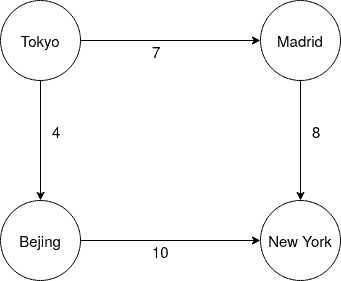In this illustration, we see a very simple shortest path problem, with “Tokyo, Bejing, New York” being the shortest path between Tokyo and New York.

Finding the shortest path can be quite costly. Imagine finding the shortest path between two cities in a navigation system, with various cities and streets between origin and target location. Dijkstra’s algorithm is a very famous method to find the shortest path since it requires very few resources. It works on every directed graph that doesn’t have negative edge valuations.

## 3. Improving Paths by Relaxing an Edge

Dijkstra’s and Bellmann Ford’s algorithm use a technique called edge relaxation. This means that during traversing our graph and finding our shortest path, we update the paths we have for already known nodes as soon as we find a shorter path to reach it. This is best illustrated in a picture: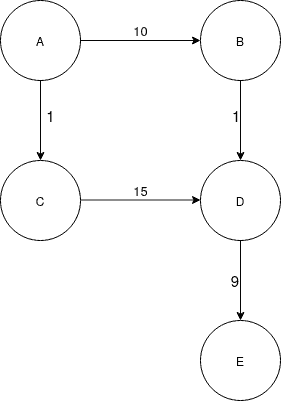In our diagram, we start at, and our target is. As we follow Dijkstra’s algorithm, we first go fromto. From, we can see that our cost to go fromtowould be 16 in total. But since Dijkstra always follows the shortest edge that has not been traversed yet, we first take the path fromto. From, we can see that pathtoonly has a cost of 11, which makes path,,cheaper than,,. This leads us to update the path toand assign it with a lower cost, 11. This process of updating a path in our graph is called relaxing the edge.

## 4. Complexity

Trying all possible paths in a graph is a superpolynomial problem. This is the reason why an efficient algorithm to find the shortest path can save us a lot of time. Dijkstra’s algorithm has a time complexity ofwhen it is implemented with a list, compared to Bellmann Ford’s algorithm with, which also uses the method of relaxing edges. Another alternative way of finding the shortest path is to find all shortest paths for every pair in the graph.

An example of an algorithm that doesn’t use relaxing edges is the Floyd algorithm. It works by calculating the shortest path between every pair of vertices available, having a complexity ofand also the benefit of calculating the shortest path in graphs with negative edges. Since Floyd’s algorithm is one of the fastest shortest path algorithms that doesn’t use edge relaxation, we can conclude that this technique gives us a huge performance increase.

## 5. Order of Relaxation

In our last paragraph, we saw that Dijkstra’s complexity differs from Bellmann Ford’s. This may be a bit surprising since they both relax edges while traversing the graph. Thus it is not only important that we relax edges but also in which order we do it. To understand this better, we have a look at the following example: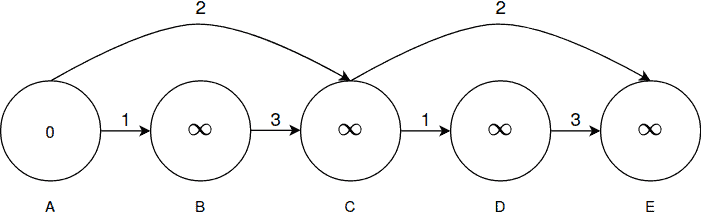First of all, we follow the path,,,,relaxing all the edges on our way: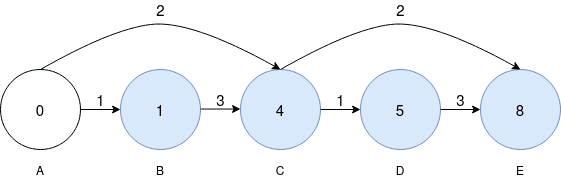Then we notice that we can relax the upper-right edge to get a shorter path to: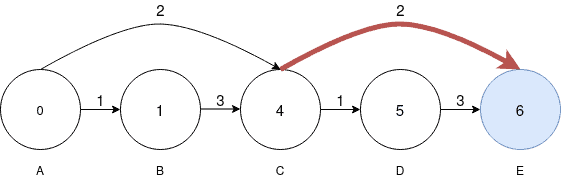After relaxing the upper-right edge, we notice that we can also relax the upper-left edge to get a shorter path to: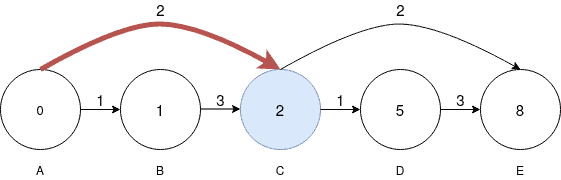Finally, we have to relax the upper-right edge again to get the shortest path of the graph.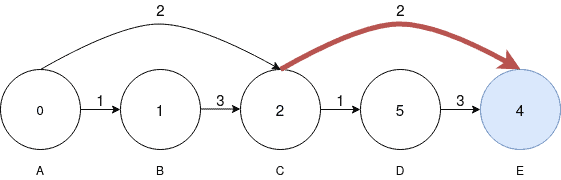As we can see, the order of relaxation matters a lot, since relaxing the upper-left edge first and then the upper-right edge after would have saved us the cost of 1 relaxation. While this doesn’t look much at first sight, the cost is actuallyfor edges of such type.

## 7. Conclusion

In this article, we discussed the concept of relaxing edges and their importance in various shortest path algorithms. Moreover, we analyzed how a different order of relaxation can result in a cost difference when calculating the shortest path.